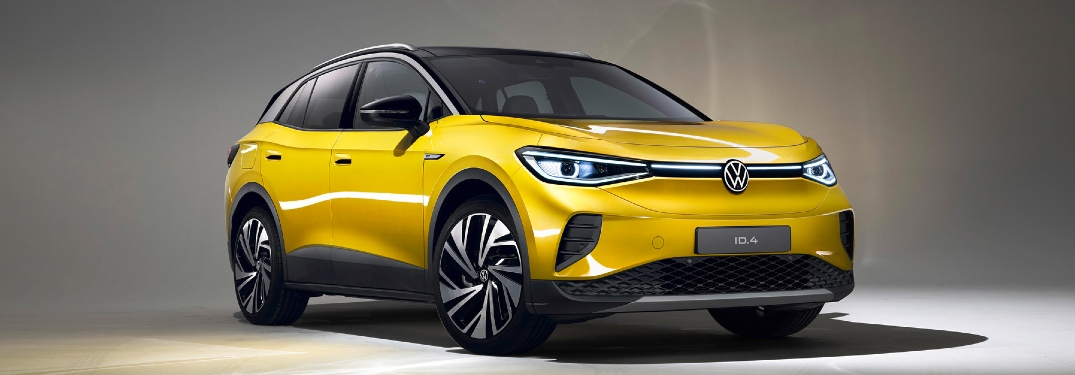## What does MPGe mean?

By Product Expert | Posted in EV, FAQs on Monday, October 26th, 2020 at 6:24 am## What is MPGe and how is it different from regular MPG?

You seen MPGe somewhere. Likely for a plug-in hybrid or all-electric vehicle. However, those numbers often seem way too big, usually over 100. Is there some weird math that artificially pumps numbers or are EVs actually that much more efficient?

What does MPGe really mean?

Reserve an VW ID.4

### MPGe Definition

Literally, MPGe is an abbreviation meaning “miles per gallon of gasoline-equivalent.” So, if a car says it has a 141 MPGe (like the Tesla Model 3), then it can go 141 miles on the electrical equivalent of one gallon of gas (which is 33.7kWh). The MPGe measurement is quantified by the EPA in kWH per 100 miles. This is unit of volume per unit of distance, which is exactly the opposite of MPG. Basically, with kWh/100mi, lower numbers are better; with MPG, higher numbers are better.

### How Much Does It Cost?

The upcoming VW ID.4 has an 87 kWh battery pack with a 250 mile range. Divide 87 kWh by 250 miles and you get .348 kWh per mile. Multiply that by 100 and you get 34.8 kWh per 100 miles. The current average electricity rate for our home state of WI is \$0.1428. So, multiply that by the 34.8 and you get \$4.9694 (which rounds up to \$4.97) to drive 100 miles. The average driver travels about 15,000 per year, putting the annual operating cost at \$745.41. For comparison, the 2020 Tiguan gets 25 combined MPG and the current average cost of gas in WI is \$1.996 per gallon. A 100-mile drive in the 2020 Tiguan takes 4 gallons and costs \$7.98. Its annual operating cost is \$1,197.60.